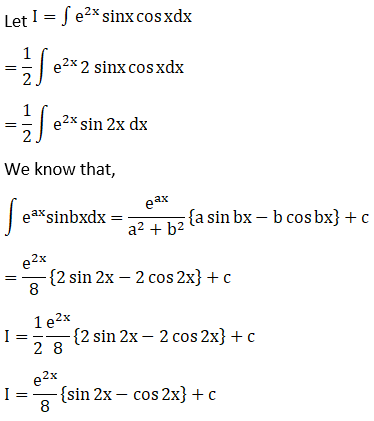# RD Sharma Solutions For Class 12 Maths Exercise 19.27 Chapter 19 Indefinite Integrals

RD Sharma Solutions for Class 12 Maths Exercise 19.27 Chapter 19 Indefinite Integrals is the best study material for students who are finding difficulties in solving problems. RD Sharma Solutions for Class 12 Maths includes all the questions provided in the textbook that is prescribed for 12th class, in accordance with the CBSE Board.

Subject experts at BYJU’S have formulated the solutions with the utmost care to help students secure good marks in their examination. This exercise deals with examining the integrals of the form

$$\begin{array}{l}\int e^{ax} \sin bx\ dx\end{array}$$
and
$$\begin{array}{l}\int e^{ax} \cos bx\ dx\end{array}$$
by using integration by parts method.

## Download the PDF of RD Sharma Solutions For Class 12 Chapter 19 – Indefinite Integrals Exercise 19.27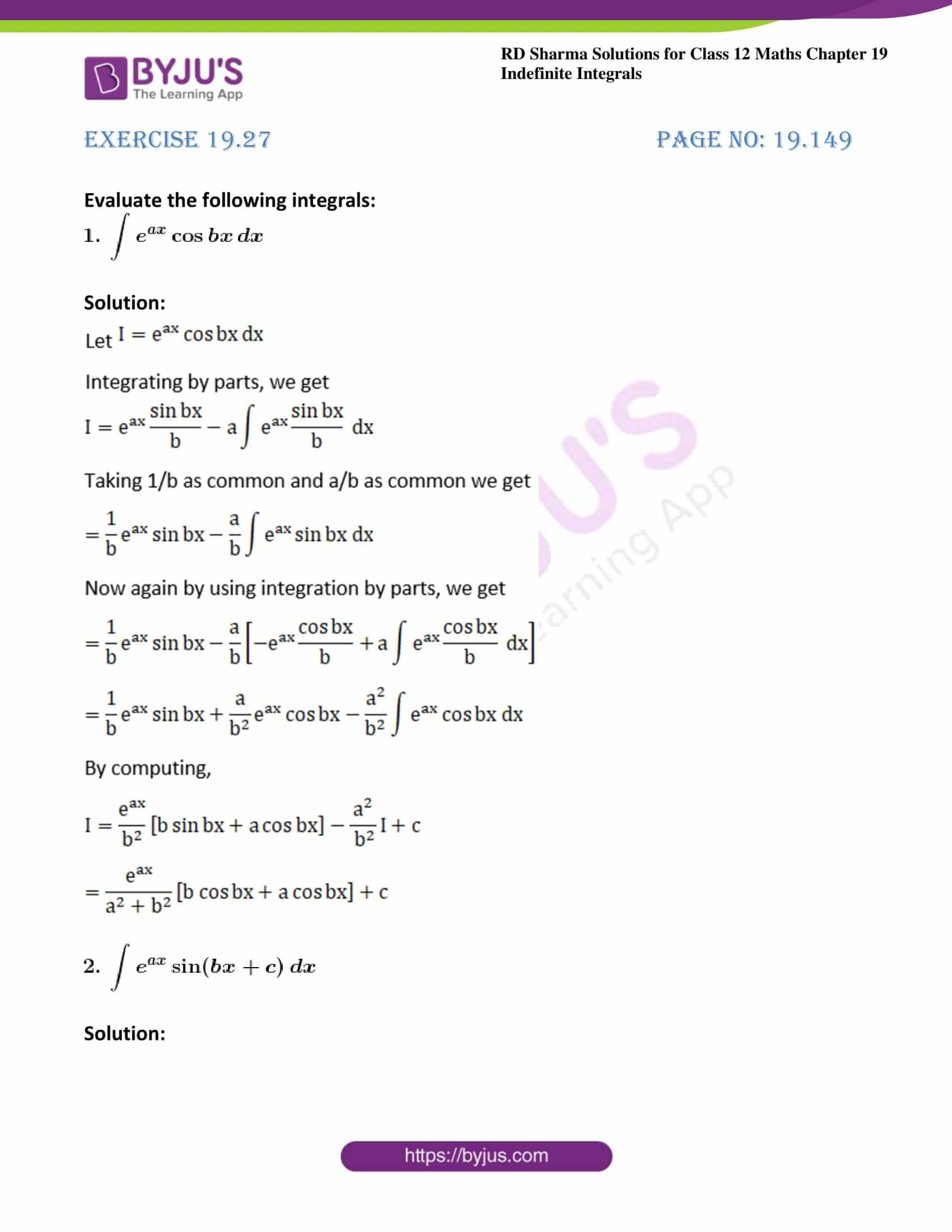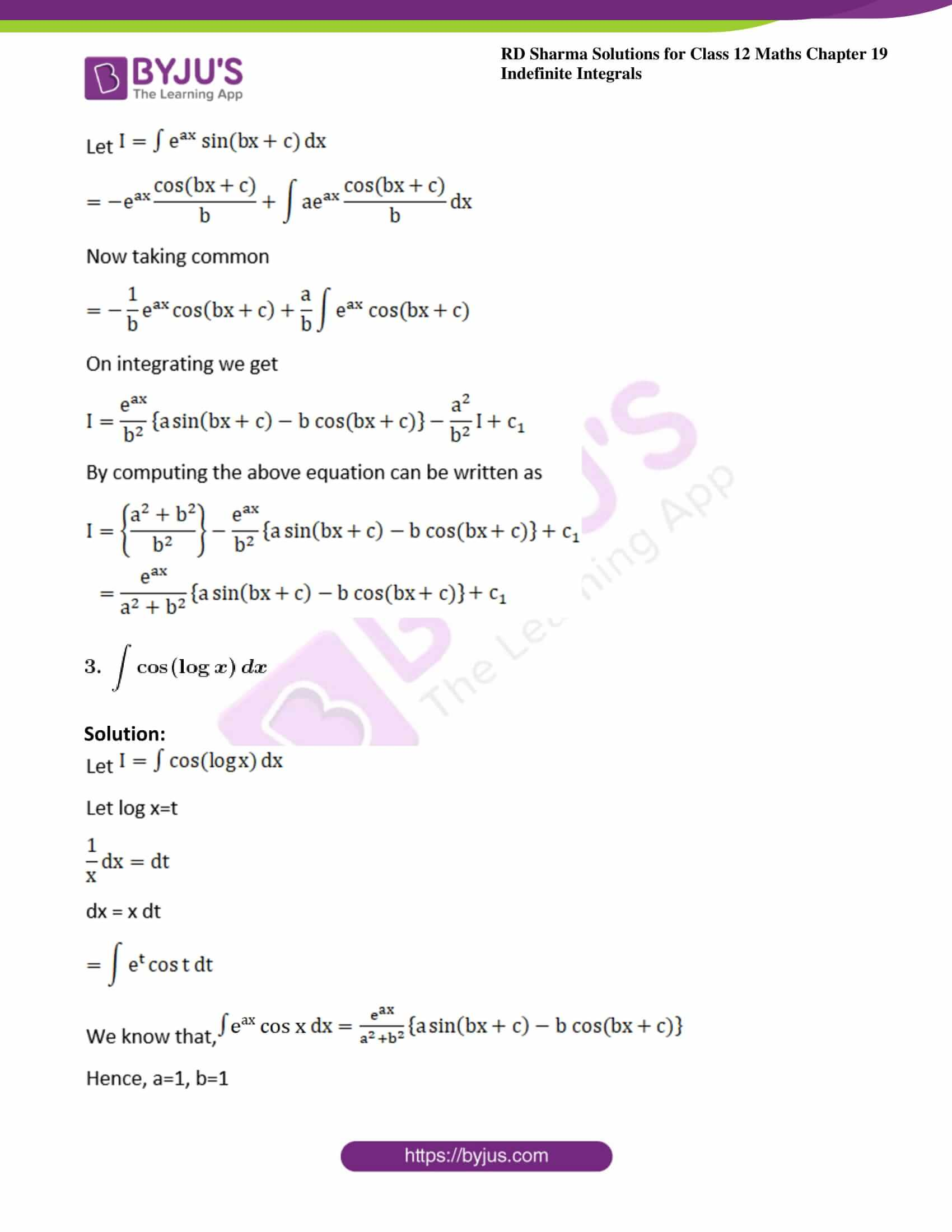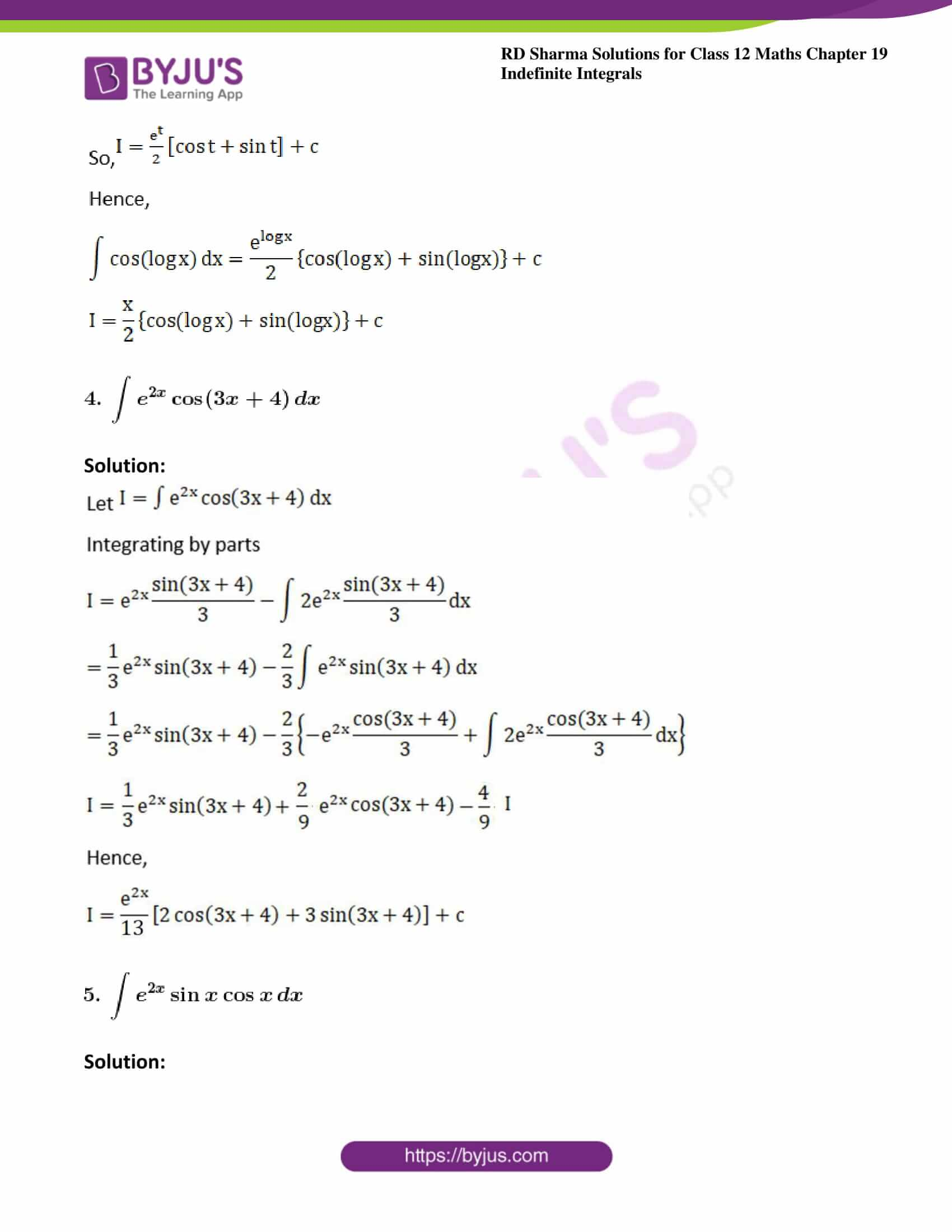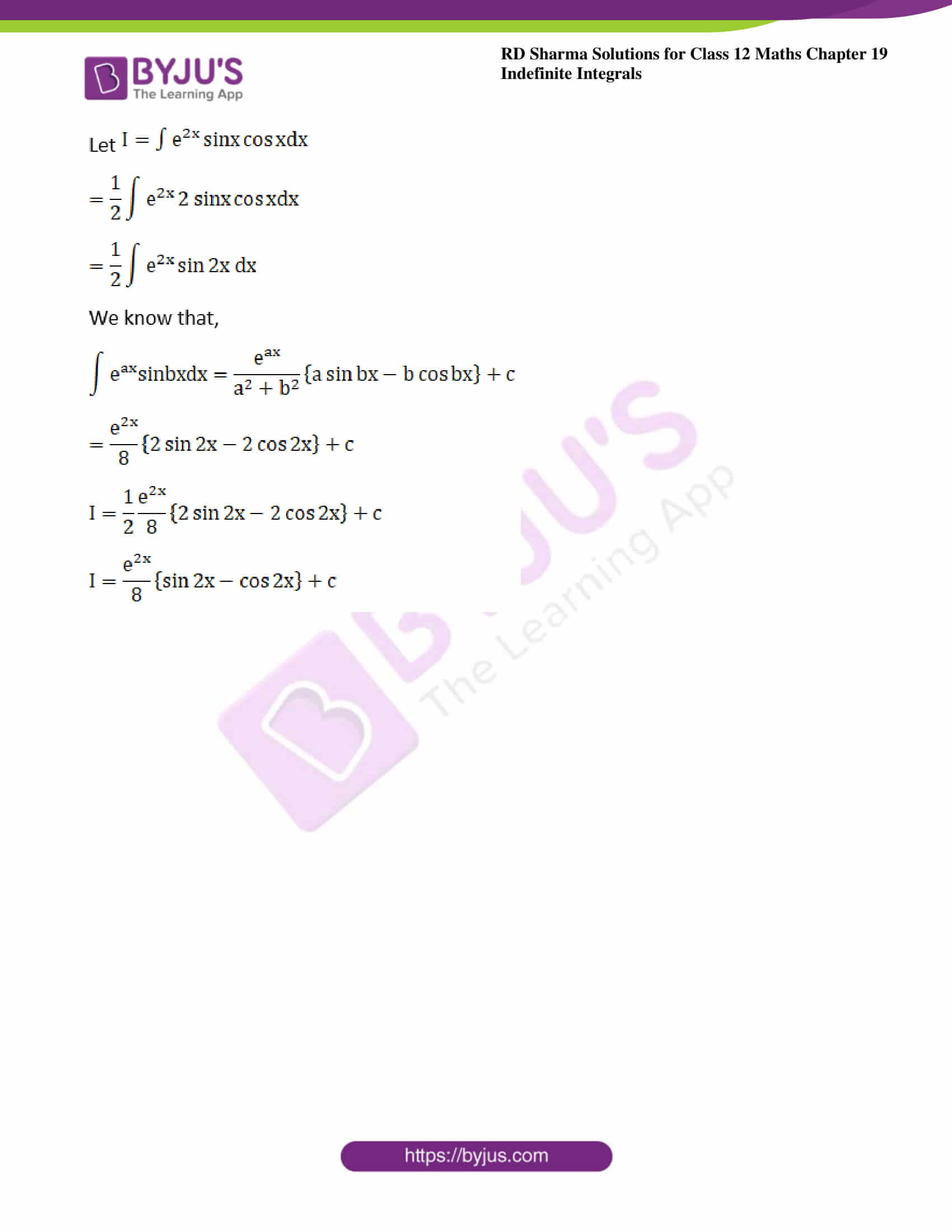### Access other exercises of RD Sharma Solutions For Class 12 Chapter 19 – Indefinite Integrals

Exercise 19.1 Solutions

Exercise 19.2 Solutions

Exercise 19.3 Solutions

Exercise 19.4 Solutions

Exercise 19.5 Solutions

Exercise 19.6 Solutions

Exercise 19.7 Solutions

Exercise 19.8 Solutions

Exercise 19.9 Solutions

Exercise 19.10 Solutions

Exercise 19.11 Solutions

Exercise 19.12 Solutions

Exercise 19.13 Solutions

Exercise 19.14 Solutions

Exercise 19.15 Solutions

Exercise 19.16 Solutions

Exercise 19.17 Solutions

Exercise 19.18 Solutions

Exercise 19.19 Solutions

Exercise 19.20 Solutions

Exercise 19.21 Solutions

Exercise 19.22 Solutions

Exercise 19.23 Solutions

Exercise 19.24 Solutions

Exercise 19.25 Solutions

Exercise 19.26 Solutions

Exercise 19.28 Solutions

Exercise 19.29 Solutions

Exercise 19.30 Solutions

Exercise 19.31 Solutions

Exercise 19.32 Solutions

### Exercise 19.27 Page No: 19.149

Evaluate the following integrals: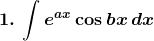Solution: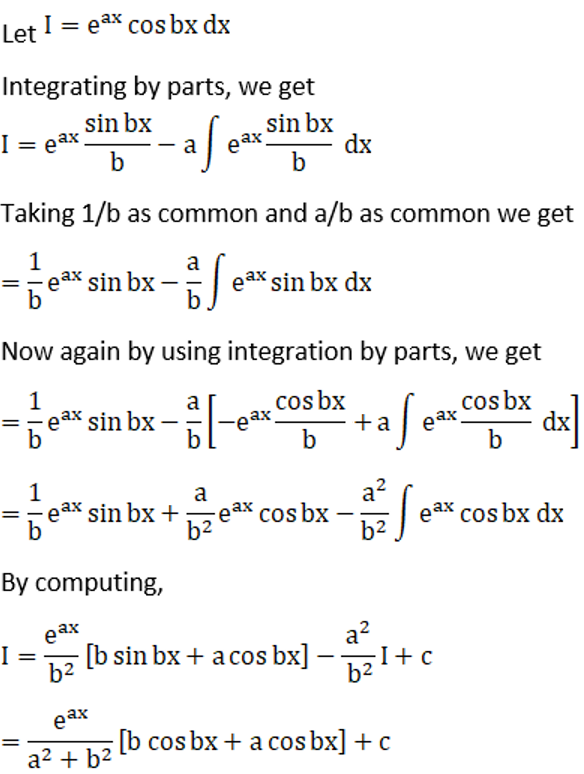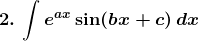Solution: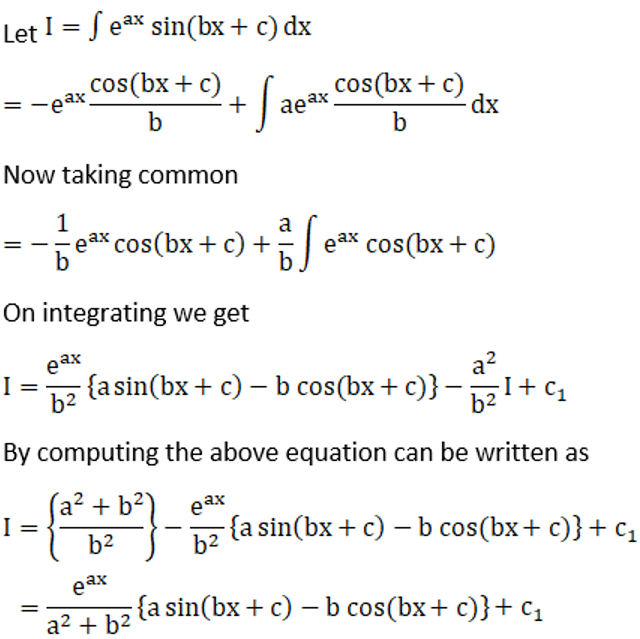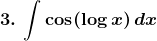Solution: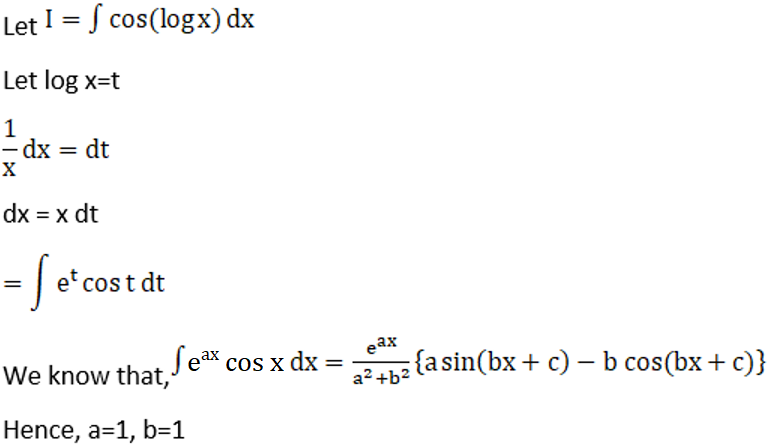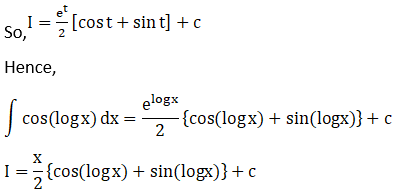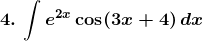Solution: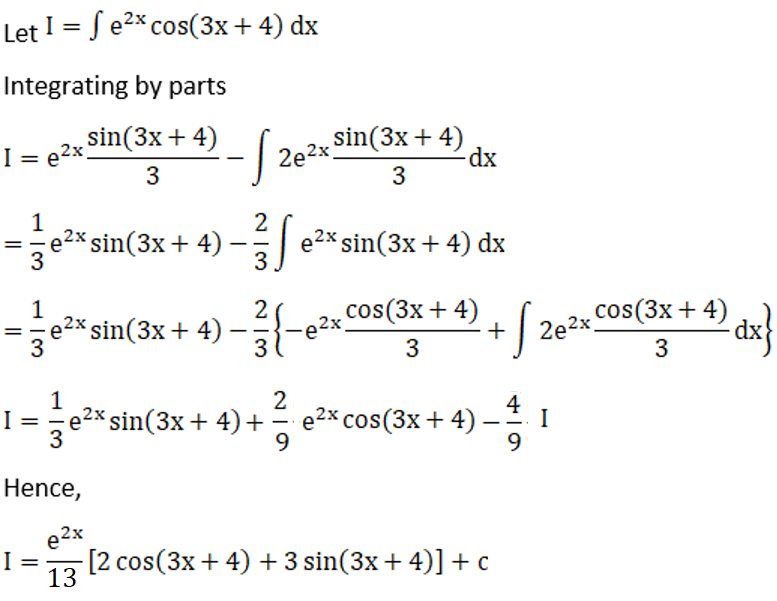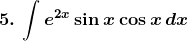Solution: